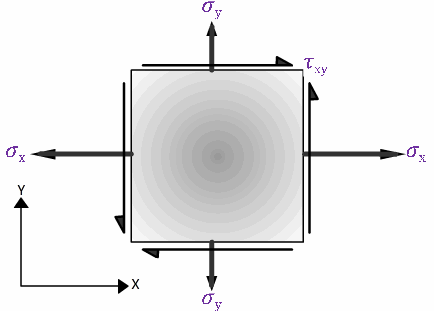# Principal Plane

1. Top view is directly over the front view.
2. Side view is inline horizontally with either top view or front view.
3. A line parallel to a plane of projection will be projected on that plane as a line.
4. A surface parallel to a plane of projection will be projected on that plane
5. A line perpendicular to a plane of projection will be projected on that plane as a point.
6. A surface perpendicular to a plane of projection will be projected on that plane as line.

Principal Plane : Concepts of principal stress and plane form backbone of material stress analysis. Purpose of this video lecture is to give you a good introduction to concept of Principal stress, Principal plane and Mohr’s circle analysis.

• Engineers most often wants to determine maximum normal stress induced at a given point for their design purpose. But there can be infinite number of planes passing through a point, and normal stress on each plane will be different from one another.
• There will be one plane on which normal stress value is maximum, this plane is known as Principal plane ( more precisely maximum principal plane) and normal stress on this plane is known as principal stress (more precisely maximum principal stress).
• Similarly there will be one more plane on which normal stress value is minimum, this is also a principal plane (minimum principal plane) and normal stress on this plane is known as Principal stress (minimum principal stress).
• 2 Dimensional Stress Analysis – Stress acting on a 2D element is shown in figure belowStress boundary conditions on a 2 dimensional element
• Mohr’s circle method is the most easy and convenient way to do stress analysis
• The procedure to draw Mohr’s circle for above case is explained below

Step1 – Draw normal and shear axes with positive axes as shownNormal and shear axes of a Mohr circle
Step2 – Mark normal stress values with sign convention, tensile stress is positive and compression stress is negativeMarking normal stress values on normal axis
Step3 – Draw shear stress values starting from already marked normal stress points.
Step4 – Connect end of shear stress linesConnecting end of shear stress lines
Step5 – Draw Mohr’s circle assuming the connection line as diameter of the circle
Step6 – Stress Analysis on Mohr circle – To get normal and shear stress values at any plane theta, take angle 2theta in Mohr circle starting from diagonal of the circle and locate a peripheral point as as shown. Shear stress value will be Y axis value and normal stress value will be X axis value.